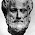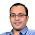## Jan 7, 2011

### Birthday Game

Source: Asked to me by Piyush (Third year Undergraduate Student, CSE, IITD)

Problem: There is a big line of people waiting outside a theater for buying tickets. The theater owner comes out and announces that the first person to have a birthday same as someone standing before him in the line gets a free ticket. Where will you stand to maximize your chance.

Solution: Solution posted by me in comments!

1.Assume line has N people. What is the prob of winning if you stand at K+1 th position ? Proportional to NcK * K. which is same as N * ( N-1cK-1). For best chances, N-1cK-1 should be maximized so K -1 should be same as (N-1)/2.
So we must stand at (N-1)/2 + 2 which is same as ceil(N/2) + 1.

2.N which was size of event space, I forgot to mention was 365. :D

So one must stand at 185th position, assuming line is long enough, else at the last position if line has less than 185 people.

3.Problem similar to a problem in a quant company selection test this year. Winning=getting free ticket
Then P(Winning)= P(Your birthday matches with someone before you AND no one else before you has matching birthdays)
Assume each persons birthday is independent. So
P(first person winning)=0
P(second person winning)= 1/365
P(third person winning)= 2/365 * 364/365
P(nth person winning) = n/365* 364/365 * ..... *(366-n)/365
Now to maximise,
P(nth person winning)>P((n-1)th person winning) and P((n+1)th person winning)
Solve to find optimal position.

4.Sorry if i get something wrong, but the goal is for me to match my birthday with somebody before me in the line, before they match their birthday.

So if I stand at the k+1th place, I can make k pairs (my date with any of the k people before me). At the same time the k people before me can make (2Ck) pairs. They will have an edge as soon as they can make more pairs than me, no?
Which happens as soon as I stand past the 3rd place.
Me in 5th pos : 4 possible date pairs.
The 4 people before me can form 6 pairs ==> They have more odds to assemble a winning pair than I have...no ?

Following that, the 3rd place is my best shot...

Where did I fail ?

5.I think the answer is approximately 20th or something. It's kind of approximate, i guess.

From this wiki page --
Birthday collision occurrence can be approximated as having a form -> p(n) = 1 - e^(-n^2/2A) where A = 365

Now, you can maximise your winning chances by maximising the function f(x) = p(x) - p(x-1); that is you cannot do any better by moving ahead in the line.

f'(x)=0 ==> 1/x = 1-(e^(-(2x-1))/2A) and putting (expected answers :P) solving gives an x = 19 or 20.

It's good to see this occurs at approx the 50% probability mark of the birthday problem where the slope of the curve is maximum.

6.Probability of winning if you stand at k+1 is P(k+1)=(binomial(n,k)*k!*k)/(n^(k+1))

P(k+1)-P(k) = positive*(k-k^2+n)

P(k+1)-P(k)<0 and P(k)-P(k-1)>0
implies
k^2-k-n>0 and k^2-3k+2-n<0

So, k>(1+sqrt(1+4n))/2 and k<(3+sqrt(1+4n))/2

n=365
k>19.61 and k<20.61

So, k=20 :)

Zubin guessed it correctly :)

7.Isn't P(k+1) = 1 - (binomial(n,k)*k!*(n-k))/(n^k+1)?

8.Aah. I missed that denominator is also a function of K and instead took it to be constant !

Anyways, its not immediately clear to me that any local maxima is also a global maxima. Any clarifications please?

9.@Nishant (Novacaine)
I am defining P(k+1) as probability that you stand at (k+1)th position and you are the first one to have a common birthday.
So, first fill first k spots with k different birthdays. then fill the last spot with any of these k entries. this gives you the numerator.

The expression you are giving gives probability = (1-probability that there is no common birthdays in first k elements) which is different from what we need to do

@Nikhil..
Global maxima has to be either local maxima or extremum points. Extremum points (standing at starting and at end) do not help. Hence, local maxima is the solution. Makes sense?

10.This comment has been removed by a blog administrator.

11.Hi Pratik,

Would you please explain how did you get this expression:

P(k+1)-P(k) = positive*(k-k^2+n)

Thanks,
Vipul

12.@Vipul..
I am assuming you understood how I got
P(k+1)=(binomial(n,k)*k!*k)/(n^(k+1))

P(k+1)=(n!*k)/(n-k)!(n^(k+1))
P(k)= (n!*(k-1))/(n-k+1)!(n^(k))

P(k+1)-P(k)= [n!/((n-k+1)!n^(k+1))]*[k(n-k+1)-(k-1)*n]
= Positive*(kn-k^2+k-kn+n) = Positive*(k+n-k^2)

Hope that clarifies.

13.Thanks a lot Pratik for explanation.

14.I am sharing my mathematical calculations here:
For the ist person ,bday =365/365
2nd person 364/365 or 1- 1/365 In case the bdays don’t collide
3rd 363/365 or 1- 2/365

And so on..
Lets assume xth position is favorable
So at xth position prob. 365-(k-1)/365 or 1- (x-1)/365

We know e^x = 1+x+x^2/2!+…..
Approx. e^x = 1+x

Therefore, 1- 1/365 = e^-1/365

1- 2/365 = e^-2/365

All are independent therefore probability for no collisions in birthdates (e^-1/365)( e^-2/365)…….( e^-(x-1)/365) =g(x)
1-g(x)=p(x) where p(x) is prob of bdays collision

f(x)=p(x)-p(x-1) it should be maximized
so we need to calculate value of f’(x) = 0

p(x)= 1- e^-(1+2+…+x-1)/365= 1- e^-(x-1)x/2*365 = 1-e^(-x^2)/2*365

p(x-1) = 1-e^(-(x-1)^2)/2*365

f(x)= e^(-(x-1)^2)/2*365 - e^(-x^2)/2*365
f’(x)= 1/x = 1-(e^(-(2x-1))/2*365)

by hit and trial I got x =19.6 or 20

then I saw http://en.wikipedia.org/wiki/Birthday_problem has the solution as 23.

15.So I tried to make a small code:

What I wrote was a recursive method.

public static void main(String[] args) {
int n;
for (n = 1; n <= 365; n++){
double k = 1- different_birthdays(n);
System.out.println( n +" "+k);
}
}
static double different_birthdays(int n)
{
return n == 1 ? 1.0 : different_birthdays(n-1) * (365.0-(n-1))/365.0;
}

}

The output of the code is coming out to be:

1 0.0
2 0.002739726027397249
3 0.008204165884781456
4 0.016355912466550326
5 0.02713557369979369
6 0.04046248364911165
7 0.05623570309597559
8 0.07433529235166925
9 0.09462383388916695
10 0.1169481777110779
11 0.14114137832173335
12 0.1670247888380647
13 0.19441027523242982
14 0.22310251200497344
15 0.2529013197636867
16 0.28360400525285023
17 0.3150076652965609
18 0.3469114178717895
19 0.37911852603153684
20 0.41143838358058016
21 0.4436883351652059
22 0.4756953076625502
23 0.5072972343239855
24 0.5383442579145289
25 0.568699703969464
26 0.598240820135939
27 0.6268592822632421
28 0.6544614723423995
29 0.680968537477777
30 0.7063162427192686
31 0.7304546337286438
32 0.7533475278503207
33 0.774971854175772
34 0.7953168646201543
35 0.8143832388747152
36 0.8321821063798795
37 0.8487340082163846
.....
at 23 position I am getting p(x)>0.5

16.Where am I wrong?

17.I got hold of it now.Here is how it goes.

When I talk that what is the minimum number of people required so that at least two people share their b’days ,its 23. Here we look for the cases where probability touches 50% for the ist time and it occurs at 23rd position. In this case we have 23C2 number of pairs to compare their respective b’days. If you put p(x)= 1-e^(-x^2)/2*365 > 0.5
You will get approx 23.
While ,in case there is a contest I chose P(x)-p(x-1) to be maximized.its nothing but mathematical form of the statement that kth person has k choices of matching bdays and also nobody has won yet who are standing in front of him.

Here no 50% thing comes,its just a simple case of finding the maxima in the curve of p(x)-p(x-1) .
In simple words for the 2nd person to win ,the conditions to be satisfied are:
1.Nobody in front of him won yet
2.he has 1 choice of dates out of 365 to match his bdays(because only one person is standing in front of him)

For kth person in queue,his winning is dependent on:
1.no body in k-1 persons standing before him won yet.
2. kth person has k-1 out of 365 to chose from ,to match his b’day.

Find the probability ,p(k)= p(no one won yet) *[(k-1)/365]

This gets maximized at 20.

18.:) Yes, you got it correct prashant. Cheers!

### Fraction Brainteaser

Source: Sent to me by Gaurav Sinha Problem: Siddhant writes a Maths test and correctly answers 5 out of 6 Arithmetic questions and 20...